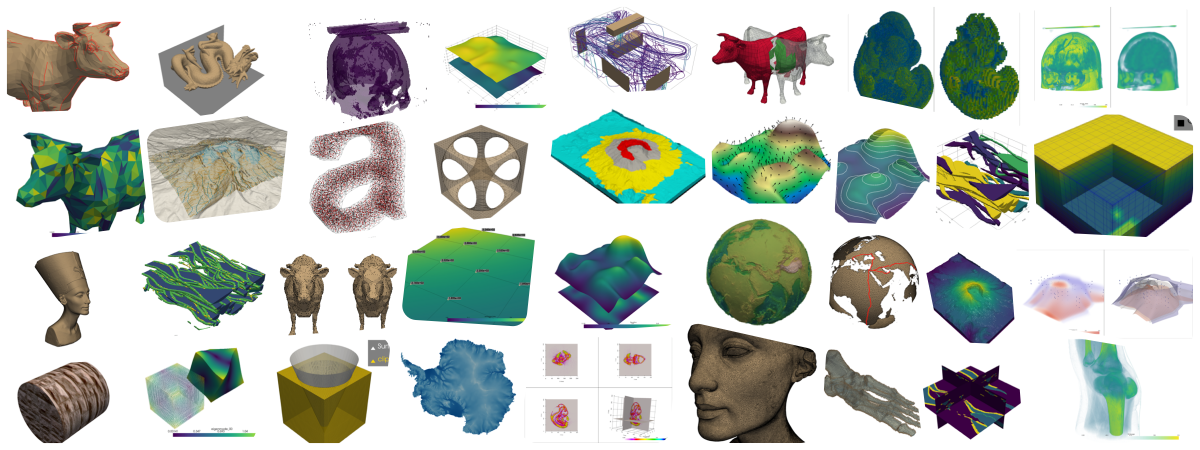# PyVista 可视化工具包 API

PyVista 正在参加 2020 年度 OSC 中国开源项目评选，请投票支持！
PyVista 在 2020 年度 OSC 中国开源项目评选 中已获得 {{ projectVoteCount }} 票，请投票支持！
PyVista 获得 2020 年度 OSC 中国开源项目评选「最佳人气项目」 !
PyVista 获得 2020 年度 OSC 中国开源项目评选「最佳人气项目」「最积极运营项目」 !
PyVista 获得 2020 年度 OSC 中国开源项目评选「最积极运营项目」 !

### 软件简介

PyVista是…

• “人类的VTK”可视化工具包（VTK）的高级API

• 空间数据集的网格数据结构和过滤方法

• 3D绘图变得简单，并为大型/复杂数据几何构建yPyVista（以前称为`vtki`）是Visualization Toolkit（VTK）的帮助程序模块，它通过NumPy和直接数组访问采用了与VTK接口不同的方法。该软件包提供了Pythonic的，文档齐全的界面，该界面公开了VTK强大的可视化后端，以促进空间参考数据集的快速原型制作，分析和可视化集成。

### 评论 (0)2020/05/23 21:52

## VTK Learning Thirty-one - pyvista

pyvista is a high-level API to the VTK 创建规则网格曲面 # sphinx_gallery_thumbnail_number = 2 import pyvista as pv import numpy as np 生成一个二维的正弦曲面 # Make data x = np.arange(-10, 10, 0.25) y = np.arange(-10, 10, 0.25) x, y = np.meshgrid(x, y) r = np.sqrt(x ** 2 + y ** 2) z = np.sin(r) # Create and plot structured grid grid = pv.StructuredGrid(x, y, z) grid.plot() # Plot mean curv...

0
0

2020/05/24 22:42

## VTK Learning Thirty-three - pyvista Geometry

Geometric Objects 绘制预定义简单几何体对象 import pyvista as pv cyl = pv.Cylinder() arrow = pv.Arrow() sphere = pv.Sphere() plane = pv.Plane() line = pv.Line() box = pv.Box() cone = pv.Cone() poly = pv.Polygon() disc = pv.Disc() p = pv.Plotter(shape=(3, 3)) # Top row p.subplot(0, 0) p.add_mesh(cyl, color="tan", show_edges=True) p.subplot(0, 1) p.add_mesh(arrow, color="tan", show_edges=True) p...

0
0

2020/05/24 19:41

0
0

2020/06/26 18:10

## PVGeo-Examples 1.1 - Using the pyvista Package

WIP: Using the pyvista Package This notebook is a work in progress to demo how PVGeo can be used with pyvista for creating integrated visualizations directly in a Python environment. At this time, the 3D rendering is perfromed in a separate window and we have yet to embed the VTK rendering windowinf into some sort of Jupyter widget. Maybe someone reading this knows how to embed the rendering wi...

0
0

2020/05/27 18:32

## VTK Learning Thirty-six- rotate polydata

pyvista 读取vtk 文件，旋转之后，并另存。 import pyvista as pv # 读取polydata mesh=pv.read("door.vtp") mesh.plot() # 绕z 轴旋转180 mesh.rotate_z(180) print(mesh) mesh.plot() # 保存旋转之后的结果 mesh.save("door.vtp") 旋转之前： 旋转之后： pyvista 旋转操作源码 def rotate_z(self, angle): """Rotate mesh about the z-axis. Parameters ---------- angle : float ...

0
0

2020/07/30 15:32

## Binder - PVGeo-Examples

0
00 评论
2 收藏